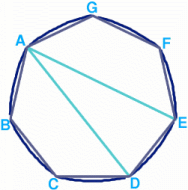#### You may also like### At a Glance

The area of a regular pentagon looks about twice as a big as the pentangle star drawn within it. Is it?### Cosines Rule

Three points A, B and C lie in this order on a line, and P is any point in the plane. Use the Cosine Rule to prove the following statement.### Six Discs

Six circular discs are packed in different-shaped boxes so that the discs touch their neighbours and the sides of the box. Can you put the boxes in order according to the areas of their bases?

# Coke Machine

##### Age 14 to 16 Challenge Level:

This is another tough nut and perhaps the diagram of the 50p piece will help.A 50 pence piece is a 7 sided polygon ABCDEFG with rounded edges, obtained by replacing the straight line DE with an arc centred at A and radius AE; replacing the straight line EF with an arc centred at B radius BF ...etc..

The 50p piece can roll in the same chute as a disc of radius $r$. Suppose the seven arcs forming the edge of the 50p piece (the arcs AB, BC etc. ) all have radius $R$ (where $R$=AD=AE=BE=BF...) then you need to find $R$ in terms of $r$. These seven arcs subtend angles of $2\pi /7$ at the centre of the disc and $2\pi /14$ at the opposite edge.